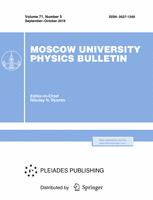Faculty of Physics
M.V.Lomonosov Moscow State University
Regular Article

# The stimulation of charge transport through the chain of semiconductor superlattices divided by the heavy doped regions

## V.A. Maksimenko$^{1,2}$, V.V. Makarov$^{1,2}$, A.E. Hramov$^{1,2}$, A.A. Koronovskii$^{1,2}$, K.N. Alekseev$^3$, A.G. Balanov$^3$

### Memoirs of the Faculty of Physics 2015. N 4.

• Article
Annotation

We proposed the mathematical model for the collective dynamics of charge in a semiconductor heterostructure which presents itself as a chain of semiconductor superlattices divided by heavy doped regions. Using the developed model we considered this structure operated in a mode characterized by the reinjection of charge domains from one superlattice to another.

PACS:
72.20.Ht High-field and nonlinear effects
05.45.-a Nonlinear dynamics and chaos
73.21.-b Electron states and collective excitations in multilayers, quantum wells, mesoscopic, and nanoscale systems
03.65.Sq Semiclassical theories and applications
Authors
V.A. Maksimenko$^{1,2}$, V.V. Makarov$^{1,2}$, A.E. Hramov$^{1,2}$, A.A. Koronovskii$^{1,2}$, K.N. Alekseev$^3$, A.G. Balanov$^3$
$^1$ Gagarin State technical university of Saratov, Saratov, 410054, Russia
$^2$ Saratov State University, Saratov, 410014, Russia
$^3$ Loughborough University, Leicestershire, UK, LE11 3TU### Moscow University Physics Bulletin### Science News of the Faculty of Physics, Lomonosov Moscow State University

This new information publication, which is intended to convey to the staff, students and graduate students, faculty colleagues and partners of the main achievements of scientists and scientific information on the events in the life of university physicists.